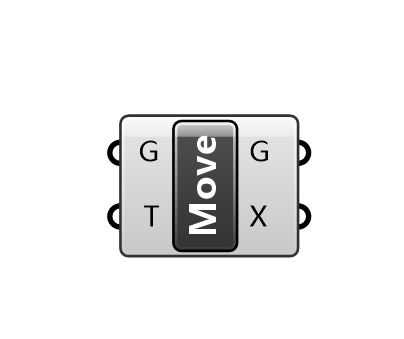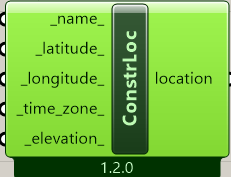# How to properly treat real world coordinates?

Hello,

I would like to know how should I prepare the data for sunlight analysis if my models have real-world coordinates and therefore are very far away from Rhino origin. I am new to Ladybug so I have spent the last two weeks on various youtube tutorials but none of them touches this topic.

I am afraid that Ladybug will perform its analysis for the building that is 9 000 kilometers from Rhino origin. What should I do to make my calculations valid?

Hey

You can change origin setting a new CPlane and set All before set it.

For the real question, I’ve made some tries, comparing a Brep in 0,0,0 and the same at 0,90000000,0.
The results wasn’t the same and the result for the far one in an broken mesh apparently, as you can see in the left simulation in image.
The Original Rhino 0,0,0 origin gave me an the middle result in image.
The mass addiction result was also different.

I haven’t tried to start my analysis with the far away geometry, starting a new grasshoper file for this test.

I would recommend to create a test file and move close to the 0,0,0 point in or closer to Sunpath object.
Regards.

If you don’t change the location of 3D model, you can use Move component in Grasshopper to move 3D model to Rhino origin（0,0,0）.@MR_Yuki is correct, always try and move the geometry to the origin.

The intuition for why this happens is that computers set limits to the amount of storage used to represent numbers. Adding the extra 9000 km to all the coordinate and vector calculations essentially uses up a lot of that storage, which means there’s less space available for preserving longer sequences of significant digits (the precision of your numbers) in the coordinates and any calculations.

That’s all you really need to know for normal use, but if you want a more explicit explanation: calculations that require preserving accuracy typically use 64 bit numbers. The 64 bits refers to the storage capacity, with each bit representing a 0 or a 1, which means numbers are represented with a base of 2 (as opposed to 10). So 3 bits with all ones (111) means each bit equals 2 by the power of the bit’s order in the sequence, multiplied by 0 or 1: 1 * 2 * 2^2 + 1 * 2 * 2^1 + 1 * 2 * 2^0 = 4 + 2 + 1 = 7 So every additional bit represents adding another power of 2 to the number.

Some of the 64 bits is used to represent where the decimal is, and if it is a positive/negative number, so the number bits dedicated to precision is actually 52. To approximate the precision you can get with this, just count the number of digits of the largest number it can represent:

2^{52 + 1} = 9007199254740992

Which is 16 significant digits. 9 km in meters (9,000,000) takes up 7 significant digits. So all your coordinate data, and calculations have 16 digits of precision when the building is at the origin, whereas the version 9000 km away has 16 - 7 = 9 digits of precision. That’s compounded in various ways and results in the accumulated error you see.

2 Likes

Also note that, in Rhino 5, all display meshes were stored as 32 bit numbers. This changed in Rhino 6 and was further improved in Rhino 7 where display meshes are stored as 64 bit numbers when necessary. Please make sure that you are always running the latest Service Release.
-wim

So to sum up: the building I want to analyze must always be in the Rhino origin 0,0,0.

But what about height? My building (and terrain around) is ~150 m above sea level. Should I take it into account or just go with normal, geographic coordinates?

I gave another try.

As far it’s close to the 0,0,0 it won’t be an issue.
I made an location and changed it’s height and also in rhino’s geo’s height and the results are the same value.
As @SaeranVasanthakumar pointed, 9000 km are too far away for.
But closer objects like 150m still has good precision.

Highland_hour_analisys.gh (41.5 KB)

Accounting for vertical distance requires a couple of additional points:

The distance above sea level won’t effect sunlight hours, and furthermore, Ladybug doesn’t use the model distance from the Rhino ground plane as a measure of distance above sea level for any simulation that does. The distance from the Rhino ground plane represents the distance to the terrain, which is an important assumption for certain simulations (i.e. to capture the logarithmic increase of wind speed with building height).

If you are running a simulation where distance above sea level is important (i.e an energy simulation) the best way to incorporate this information is by selecting a weather file from a location with a sea level within 100m of your site’s sea level (and a distance 30 - 50 km) so that the sea level impact is captured in your other environmental variables (i.e air pressure).

So in summary, even for simulations where this won’t matter (i.e the sunlight hours analysis) I recommend moving the building down to avoid breaking this assumption, and to capture better accuracy in modeling. For simulations using numerical methods (i.e energy simulations, the UWG) this is particularly important since initial states are accumulated at each time step and you can end up with numbers that exceed 64 bits.

Slightly off tangent: some of this could probably be incorporated into the tools. For example, I incorporated rescaling and recentering for the geometric inputs of the wind rose so that the same precision is maintained regardless of where it is in Rhino. The straight skeleton algorithm used in the perimeter-core splitting component is actually a little unstable right now because it needs something like this, but I didn’t incorporate it while I was writing the code because it needs a more calculated combination of rescaling, recentering and possibly division by its standard deviation.

1 Like

Fascinating. I finally have an explanation for why the meshes are the first to go crazy when the analysis moves away from the origin.

@wim, how does Rhino 7 decide when to use 64 vs 32 bit numbers?

Thank you once again for such valuable information.
Since I can no longer rely on my survey coordinates I have to deliver the location the other way. I think I will use “Construct location”The nearest epw file is from the city 25 km afar. Should I use this file or it is better to construct location and put there the direct location of the centre of the building?